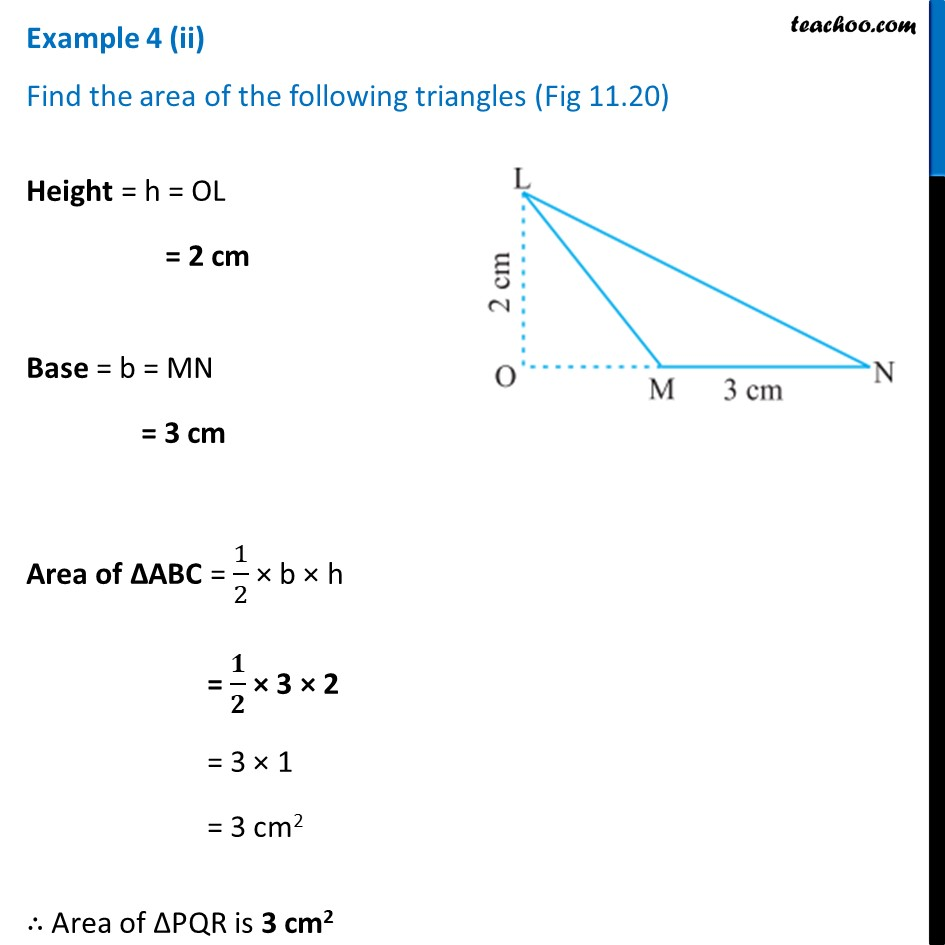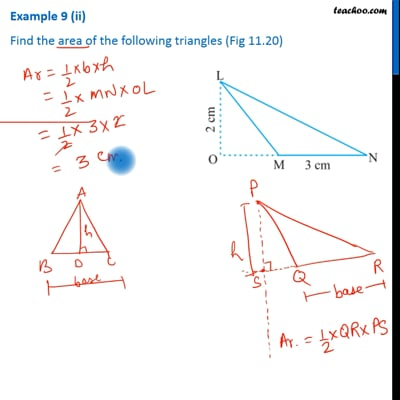Examples

Chapter 9 Class 7 Perimeter and Area
Serial order wiseThis video is only available for Teachoo black users

Learn in your speed, with individual attention - Teachoo Maths 1-on-1 Class

### Transcript

Example 4 (ii) Find the area of the following triangles (Fig 11.20) Height = h = OL = 2 cm Base = b = MN = 3 cm Area of ∆ABC = 1/2 × b × h = 𝟏/𝟐 × 3 × 2 = 3 × 1 = 3 cm2 ∴ Area of ∆PQR is 3 cm2